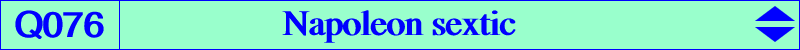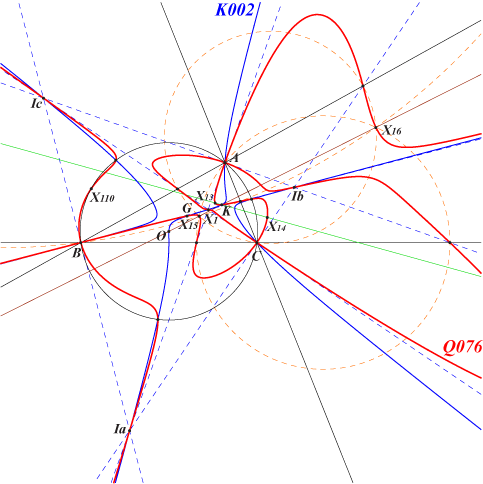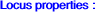see belowX(1), X(2), X(6), X(13), X(14), X(15), X(16), X(110), X(523) excenters, feet of bisectors points of the Thomson cubic K002 at infinity and on the circumcircle of ABC i.e. vertices ofQ076 is a circular isogonal sextic, a member of the pencil generated by Q039 and Q075. The equation of Q076 can be written under the form : xyz ∑(a2 SA+2 SB SC) x (c2y2- b2z2) + ∏(c2y2- b2z2) = 0, where ∑(a2 SA+2 SB SC) x (c2y2- b2z2) = 0 is the equation of the Napoleon cubic K005 and ∏(c2y2- b2z2) = 0 is that of the six bisectors of ABC. It follows that the tangents at the in/excenters to Q076 pass through the nine point center X(5) of ABC.The GK line (when defined) of a triangle is the line passing through its centroid and its symmedian point. Let P be a point. Denote by Bc the intersection of AB with the parallel line to AC through P, by Cb the intersection of AC with the parallel line to AB through P. The other intersections Ca, Ac, Ab and Ba are defined cyclically. The GK lines of PBcCb, PCaAc and PAbBa concur if and only if P lies on the Napoleon sextic Q076 (Angel Montesdeoca 2014-09-28, ADGEOM #1853). If the GK line is replaced with the Euler line, the locus is K502.